Anúncio

# Beams design and analysis

--
20 de Aug de 2016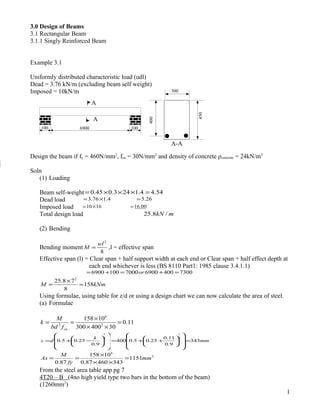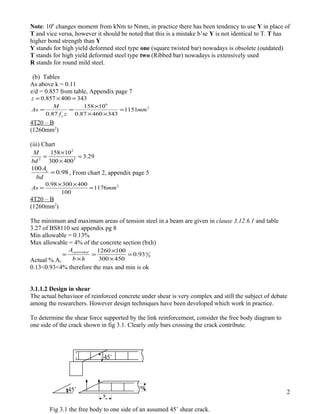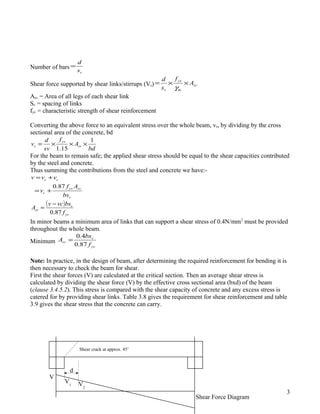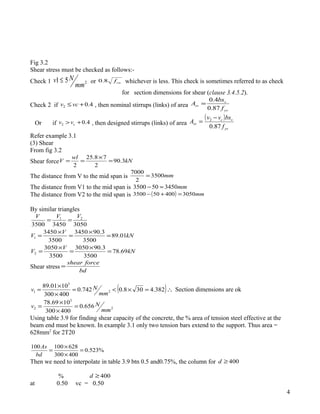Anúncio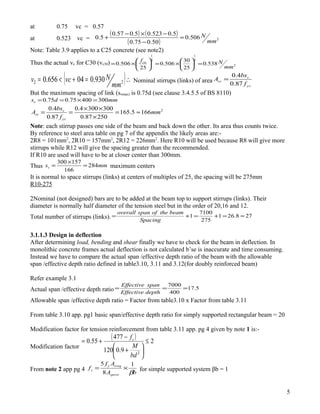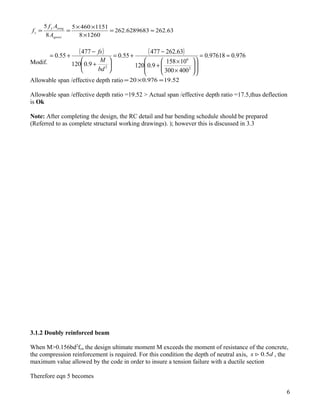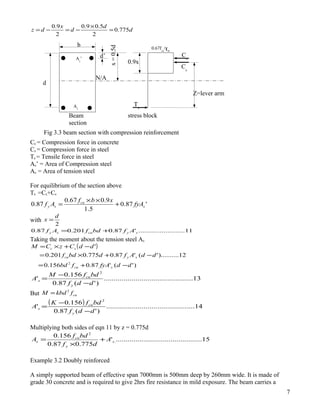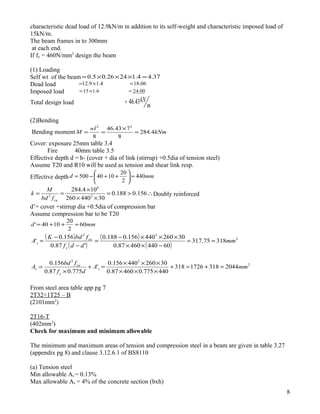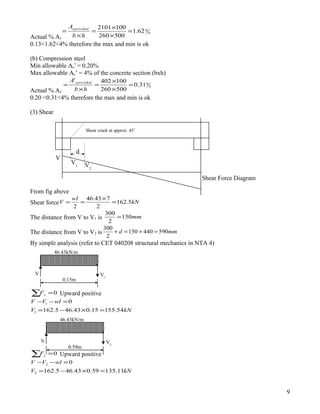Anúncio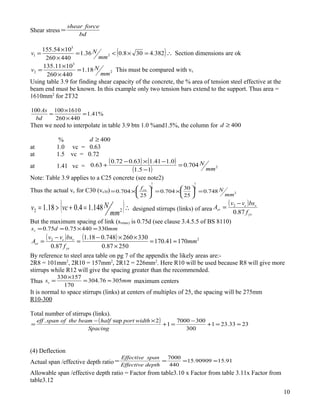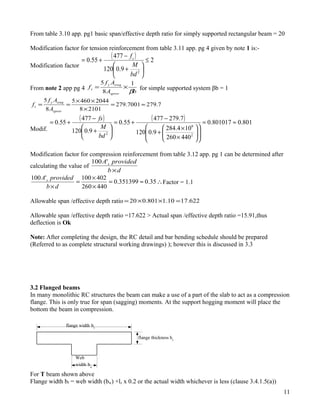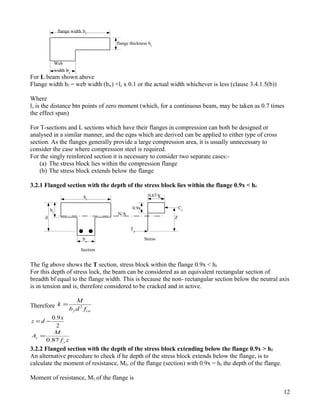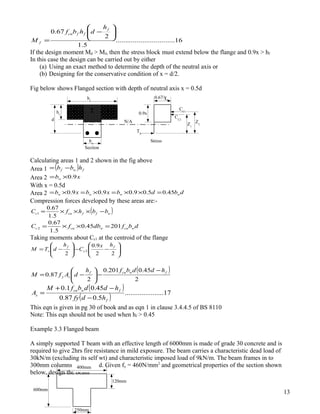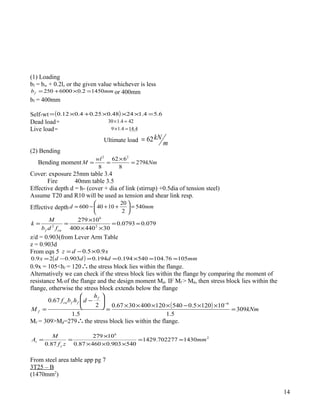Anúncio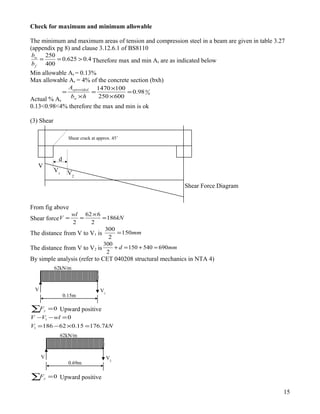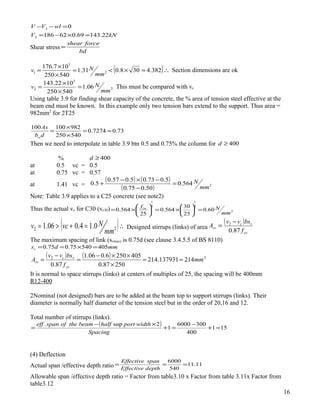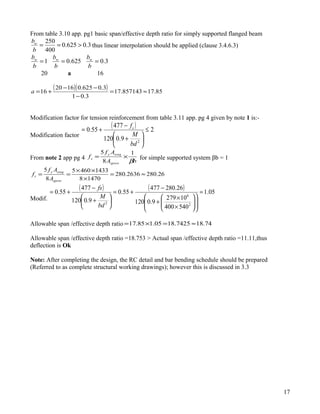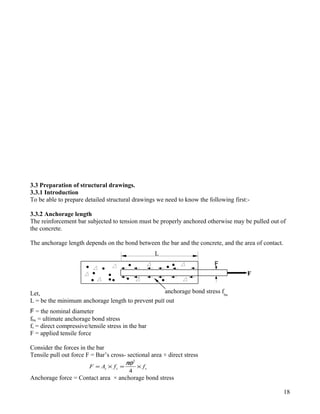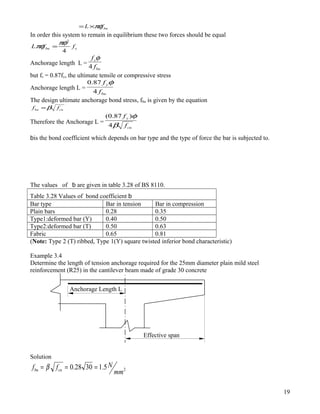Anúncio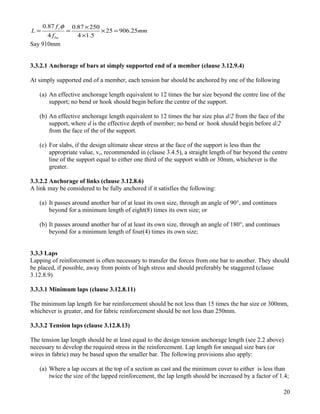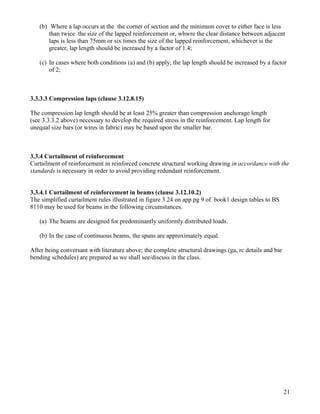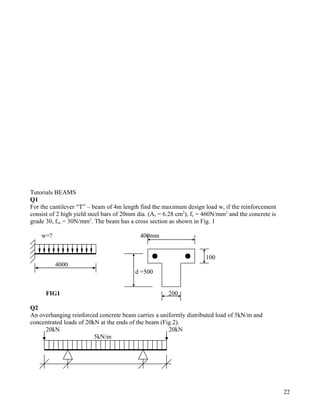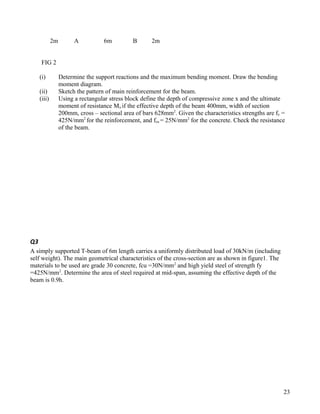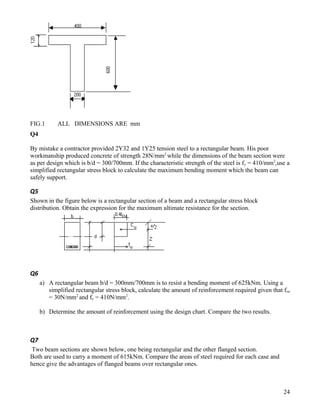Anúncio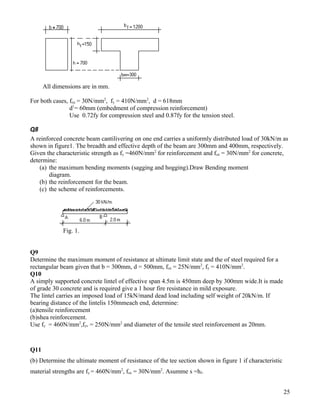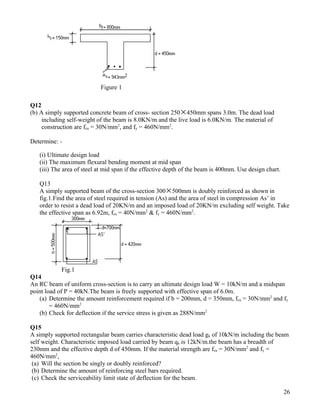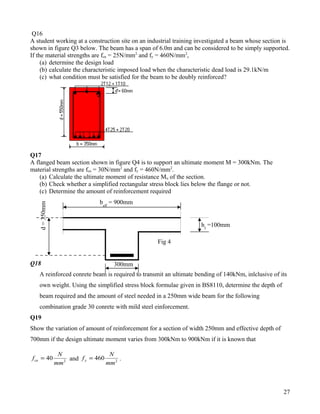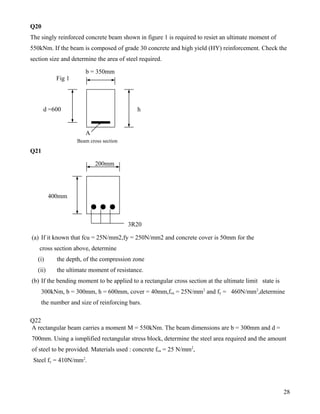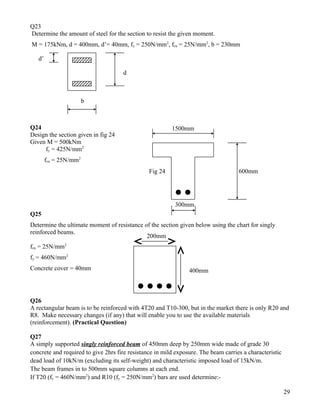Anúncio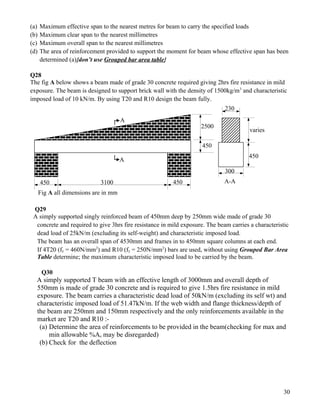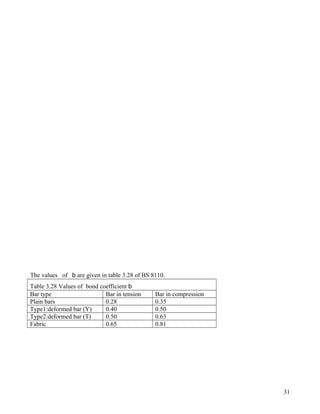Próximos SlideShares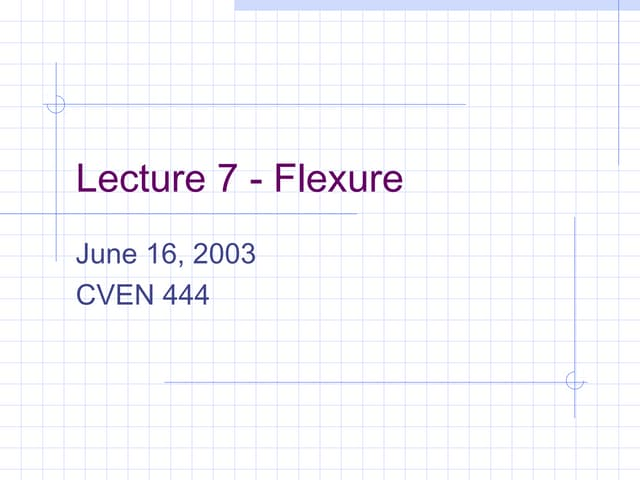Analysis of T-Beam
Carregando em ... 3
1 de 31
Anúncio

### Beams design and analysis

1. 3.0 Design of Beams 3.1 Rectangular Beam 3.1.1 Singly Reinforced Beam Example 3.1 Uniformly distributed characteristic load (udl) Dead = 3.76 kN/m (excluding beam self weight) Imposed = 10kN/m Design the beam if fy = 460N/mm2 , fcu = 30N/mm2 and density of concrete ρconcrete = 24kN/m3 Soln (1) Loading Beam self-weight 54.44.1243.045.0 =×××= Dead load 26.54.176.3 =×= Imposed load 00.161610 =×= Total design load mkN /8.25 (2) Bending Bending moment 8 2 wl M = ,l = effective span Effective span (l) = Clear span + half support width at each end or Clear span + half effect depth at each end whichever is less (BS 8110 Part1: 1985 clause 3.4.1.1) 7300400690070001006900 =+=+= or kNmM 158 8 78.25 2 = × = Using formulae, using table for z/d or using a design chart we can now calculate the area of steel. (a) Formulae 11.0 30400300 10158 2 6 2 = ×× × == cufbd M k                 −+= 2 1 9.0 25.05.0 k dz mm343 9.0 11.0 25.05.0400 2 1 =                 ++= 2 6 1151 34346087.0 10158 87.0 mm fy M As = ×× × == From the steel area table app pg 7 4T20 – B (4no high yield type two bars in the bottom of the beam) (1260mm2 ) 1 A A A-A 450 1006900100 300 400
2. Note: 106 changes moment from kNm to Nmm, in practice there has been tendency to use Y in place of T and vice versa, however it should be noted that this is a mistake b’se Y is not identical to T. T has higher bond strength than Y Y stands for high yield deformed steel type one (square twisted bar) nowadays is obsolete (outdated) T stands for high yield deformed steel type two (Ribbed bar) nowadays is extensively used R stands for round mild steel. (b) Tables As above k = 0.11 z/d = 0.857 from table, Appendix page 7 343400857.0 =×=z 2 6 1151 34346087.0 10158 87.0 mm zf M As y = ×× × == 4T20 – B (1260mm2 ) (iii) Chart 29.3 400300 10158 2 2 2 = × × = bd M 98.0 100 = bd As , From chart 2, appendix page 5 2 1176 100 40030098.0 mmAs = ×× = 4T20 – B (1260mm2 ) The minimum and maximum areas of tension steel in a beam are given in clause 3.12.6.1 and table 3.27 of BS8110 see appendix pg 8 Min allowable = 0.13% Max allowable = 4% of the concrete section (bxh) Actual % As 0 093.0 450300 1001260 = × × = × = hb A provideds 0.13<0.93<4% therefore the max and min is ok 3.1.1.2 Design in shear The actual behaviuor of reinforced concrete under shear is very complex and still the subject of debate among the researchers. However design techniques have been developed which work in practice. To determine the shear force supported by the link reinforcement, consider the free body diagram to one side of the crack shown in fig 3.1. Clearly only bars crossing the crack contribute. 2 sv d Fig 3.1 the free body to one side of an assumed 45˚ shear crack. 45˚ 45˚
3. Number of bars vs d = Shear force supported by shear links/stirrups (Vs) sv m yv v A f s d ××= γ Asv = Area of all legs of each shear link Sv = spacing of links fyv = characteristic strength of shear reinforcement Converting the above force to an equivalent stress over the whole beam, vs, by dividing by the cross sectional area of the concrete, bd bd A f sv d v sv yv s 1 15.1 ×××= For the beam to remain safe; the applied shear stress should be equal to the shear capacities contributed by the steel and concrete. Thus summing the contributions from the steel and concrete we have:- v svyv c cs bs Af v vvv 87.0 += += ( ) yv v sv f bsvcv A 87.0 − = In minor beams a minimum area of links that can support a shear stress of 0.4N/mm2 must be provided throughout the whole beam. Minimum yv v sv f bs A 87.0 4.0 = Note: In practice, in the design of beam, after determining the required reinforcement for bending it is then necessary to check the beam for shear. First the shear forces (V) are calculated at the critical section. Then an average shear stress is calculated by dividing the shear force (V) by the effective cross sectional area (bxd) of the beam (clause 3.4.5.2). This stress is compared with the shear capacity of concrete and any excess stress is catered for by providing shear links. Table 3.8 gives the requirement for shear reinforcement and table 3.9 gives the shear stress that the concrete can carry. 3 V2 V1 Shear Force Diagram d V Shear crack at approx. 45˚
4. Fig 3.2 Shear stress must be checked as follows:- Check 1 251 mm Nv ≤ or cuf8.0 whichever is less. This check is sometimes referred to as check for section dimensions for shear (clause 3.4.5.2). Check 2 if 4.02 +≤ vcv , then nominal stirrups (links) of area yv v sv f bs A 87.0 4.0 = Or if 4.02 +> cvv , then designed stirrups (links) of area ( ) yv vc sv f bsvv A 87.0 2 − = Refer example 3.1 (3) Shear From fig 3.2 Shear force kN wl V 3.90 2 78.25 2 = × == The distance from V to the mid span is mm3500 2 7000 = The distance from V1 to the mid span is mm3450503500 =− The distance from V2 to the mid span is ( ) mm3050400503500 =+− By similar triangles kN V V kN V V VVV 69.78 3500 3.903050 3500 3050 01.89 3500 3.903450 3500 3450 305034503500 2 1 21 = × = × = = × = × = == Shear stress bd forceshear = ( )∴=×<= × × = 382.4308.0742.0 400300 1001.89 2 3 1 mm Nv Section dimensions are ok 2 3 2 656.0 400300 1069.78 mm Nv = × × = Using table 3.9 for finding shear capacity of the concrete, the % area of tension steel effective at the beam end must be known. In example 3.1 only two tension bars extend to the support. Thus area = 628mm2 for 2T20 %523.0 400300 628100100 = × × = bd As Then we need to interpolate in table 3.9 btn 0.5 and0.75%, the column for 400≥d % 400≥d at 0.50 vc = 0.50 4
5. at 0.75 vc = 0.57 at 0.523 vc = ( ) ( ) ( ) 2506.0 50.075.0 5.0523.05.057.0 5.0 mm N= − −×− + Note: Table 3.9 applies to a C25 concrete (see note2) Thus the actual vc for C30 (vc30) 2 3 1 3 1 538.0 25 30 506.0 25 506.0 mm Nfcu =      ×=      ×= ( )∴=+<= 22 930.004656.0 mm Nvcv Nominal stirrups (links) of area yv v sv f bs A 87.0 4.0 = But the maximum spacing of link (svmax) is 0.75d (see clause 3.4.5.5 of BS 8110) mmdsv 30040075.075.0 =×== 2 1665.165 25087.0 3003004.0 87.0 4.0 mm f bs A yv v sv ≈= × ×× == Note: each stirrup passes one side of the beam and back down the other. Its area thus counts twice. By reference to steel area table on pg 7 of the appendix the likely areas are:- 2R8 = 101mm2 , 2R10 = 157mm2 , 2R12 = 226mm2 . Here R10 will be used because R8 will give more stirrups while R12 will give the spacing greater than the recommended. If R10 are used will have to be at closer center than 300mm. Thus mmsv 284 166 157300 = × = maximum centers It is normal to space stirrups (links) at centers of multiples of 25, the spacing will be 275mm R10-275 2Nominal (not designed) bars are to be added at the beam top to support stirrups (links). Their diameter is normally half diameter of the tension steel but in the order of 20,16 and 12. Total number of stirrups (links). 278.261 275 7100 1 ≈=+=+= Spacing beamtheofspanoverall 3.1.1.3 Design in deflection After determining load, bending and shear finally we have to check for the beam in deflection. In monolithic concrete frames actual deflection is not calculated b’se is inaccurate and time consuming. Instead we have to compare the actual span /effective depth ratio of the beam with the allowable span /effective depth ratio defined in table3.10, 3.11 and 3.12(for doubly reinforced beam) Refer example 3.1 Actual span /effective depth ratio 5.17 400 7000 === depthEffective spanEffective Allowable span /effective depth ratio = Factor from table3.10 x Factor from table 3.11 From table 3.10 app. pg1 basic span/effective depth ratio for simply supported rectangular beam = 20 Modification factor for tension reinforcement from table 3.11 app. pg 4 given by note 1 is:- Modification factor ( ) 2 9.0120 477 55.0 2 ≤       + − += bd M fs From note 2 app pg 4 bA Af f sprov sreqy s β 1 8 5 ×= for simple supported system βb = 1 5
6. 63.2626289683.262 12608 11514605 8 5 ≈= × ×× == sprov sreqy s A Af f Modif. ( ) ( ) 976.097618.0 400300 10158 9.0120 63.262477 55.0 9.0120 477 55.0 2 6 2 ≈=               × × + − +=       + − += bd M fs Allowable span /effective depth ratio 52.19976.020 =×= Allowable span /effective depth ratio =19.52 > Actual span /effective depth ratio =17.5,thus deflection is Ok Note: After completing the design, the RC detail and bar bending schedule should be prepared (Referred to as complete structural working drawings). ); however this is discussed in 3.3 3.1.2 Doubly reinforced beam When M>0.156bd2 fcu the design ultimate moment M exceeds the moment of resistance of the concrete, the compression reinforcement is required. For this condition the depth of neutral axis, dx 5.0 , the maximum value allowed by the code in order to insure a tension failure with a ductile section Therefore eqn 5 becomes 6
7. d d d x dz 775.0 2 5.09.0 2 9.0 = × −=−= Cc = Compression force in concrete Cs = Compression force in steel Ts = Tensile force in steel As’ = Area of Compression steel As = Area of tension steel For equilibrium of the section above Ts =Cc+Cs '87.0 5.1 9.067.0 87.0 s cu sy fyA xbf Af + ×× = with 2 d x = 11........................'87.0201.087.0 sycusy AfbdfAf += Taking the moment about the tension steel As ( ) )'('87.0156.0 12.).........'('87.0775.0201.0 ' 2 ddfyAfbd ddAfdbdf ddCzCM scu sycu sc −+= −+×= −+×= 13............................................. )'(87.0 156.0 ' 2 ddf bdfM A y cu s − − = But cufkbdM 2 = ( ) 14............................................. )'(87.0 156.0 ' 2 ddf bdfK A y cu s − − = Multiplying both sides of eqn 11 by z = 0.775d 15............................................' 775.087.0 156.0 . 2 s y cu s A df bdf A + × = Example 3.2 Doubly reinforced A simply supported beam of effective span 7000mm is 500mm deep by 260mm wide. It is made of grade 30 concrete and is required to give 2hrs fire resistance in mild exposure. The beam carries a 7 Z=lever arm Cs 0.67fcu /ɣm d’ b Beam section stress blockx=0.5d 0.9x d N/A Fig 3.3 beam section with compression reinforcement As ’ Ts Cc As
9. Actual % As 0 062.1 500260 1002101 = × × = × = hb A provideds 0.13<1.62<4% therefore the max and min is ok (b) Compression steel Min allowable As’ = 0.20% Max allowable As’ = 4% of the concrete section (bxh) Actual % As’ 0 031.0 500260 100402' = × × = × = hb A provideds 0.20 <0.31<4% therefore the max and min is ok (3) Shear From fig above Shear force kN wl V 5.162 2 743.46 2 = × == The distance from V to V1 is mm150 2 300 = The distance from V to V2 is mmd 590440150 2 300 =+=+ By simple analysis (refer to CET 040208 structural mechanics in NTA 4) ∑ =0yF Upward positive kNV wlVV 54.15515.043.465.162 0 1 1 =×−= =−− ∑ =0yF Upward positive kNV wlVV 11.13559.043.465.162 0 2 2 =×−= =−− 9 V V1 0.15m 46.43kN/m V V2 0.59m 46.43kN/m V2 V1 Shear Force Diagram d V Shear crack at approx. 45˚
10. Shear stress bd forceshear = ( )∴=×<= × × = 382.4308.036.1 440260 1054.155 2 3 1 mm Nv Section dimensions are ok 2 3 2 18.1 440260 1011.135 mm Nv = × × = This must be compared with vc Using table 3.9 for finding shear capacity of the concrete, the % area of tension steel effective at the beam end must be known. In this example only two tension bars extend to the support. Thus area = 1610mm2 for 2T32 %41.1 440260 1610100100 = × × = bd As Then we need to interpolate in table 3.9 btn 1.0 %and1.5%, the column for 400≥d % 400≥d at 1.0 vc = 0.63 at 1.5 vc = 0.72 at 1.41 vc = ( ) ( ) ( ) 2704.0 15.1 0.141.163.072.0 63.0 mm N= − −×− + Note: Table 3.9 applies to a C25 concrete (see note2) Thus the actual vc for C30 (vc30) 2 3 1 3 1 748.0 25 30 704.0 25 704.0 mm Nfcu =      ×=      ×= ( )∴=+>= 22 148.14.018.1 mm Nvcv designed stirrups (links) of area ( ) yv vc sv f bsvv A 87.0 2 − = But the maximum spacing of link (svmax) is 0.75d (see clause 3.4.5.5 of BS 8110) mmdsv 33044075.075.0 =×== ( ) ( ) 22 17041.170 25087.0 330260748.018.1 87.0 mm f bsvv A yv vc sv ≈= × ××− = − = By reference to steel area table on pg 7 of the appendix the likely areas are:- 2R8 = 101mm2 , 2R10 = 157mm2 , 2R12 = 226mm2 . Here R10 will be used because R8 will give more stirrups while R12 will give the spacing greater than the recommended. Thus mmsv 30576.304 170 157330 ≈= × = maximum centers It is normal to space stirrups (links) at centers of multiples of 25, the spacing will be 275mm R10-300 Total number of stirrups (links). ( ) 2333.231 300 3007000 1 2sup. ≈=+ − =+ ×− = Spacing widthporthalfbeamtheofspaneff (4) Deflection Actual span /effective depth ratio 91.1590909.15 440 7000 ≈=== depthEffective spanEffective Allowable span /effective depth ratio = Factor from table3.10 x Factor from table 3.11x Factor from table3.12 10
11. From table 3.10 app. pg1 basic span/effective depth ratio for simply supported rectangular beam = 20 Modification factor for tension reinforcement from table 3.11 app. pg 4 given by note 1 is:- Modification factor ( ) 2 9.0120 477 55.0 2 ≤       + − += bd M fs From note 2 app pg 4 bA Af f sprov sreqy s β 1 8 5 ×= for simple supported system βb = 1 7.2797001.279 21018 20444605 8 5 ≈= × ×× == sprov sreqy s A Af f Modif. ( ) ( ) 801.0801017.0 440260 104.284 9.0120 7.279477 55.0 9.0120 477 55.0 2 6 2 ≈=               × × + − +=       + − += bd M fs Modification factor for compression reinforcement from table 3.12 app. pg 1 can be determined after calculating the value of db providedA s × '100 ∴≈= × × = × 35.0351399.0 440260 402100'100 db providedA s Factor = 1.1 Allowable span /effective depth ratio 622.1710.1801.020 =××= Allowable span /effective depth ratio =17.622 > Actual span /effective depth ratio =15.91,thus deflection is Ok Note: After completing the design, the RC detail and bar bending schedule should be prepared (Referred to as complete structural working drawings) ); however this is discussed in 3.3 3.2 Flanged beams In many monolithic RC structures the beam can make a use of a part of the slab to act as a compression flange. This is only true for span (sagging) moments. At the support hogging moment will place the bottom the beam in compression. For T beam shown above Flange width bf = web width (bw) +lz x 0.2 or the actual width whichever is less (clause 3.4.1.5(a)) 11 flange width bf flange thickness hf Web width bw
12. For L beam shown above Flange width bf = web width (bw) +lz x 0.1 or the actual width whichever is less (clause 3.4.1.5(b)) Where lz is the distance btn points of zero moment (which, for a continuous beam, may be taken as 0.7 times the effect span) For T-sections and L sections which have their flanges in compression can both be designed or analysed in a similar manner, and the eqns which are derived can be applied to either type of cross section. As the flanges generally provide a large compression area, it is usually unnecessary to consider the case where compression steel is required. For the singly reinforced section it is necessary to consider two separate cases:- (a) The stress block lies within the compression flange (b) The stress block extends below the flange 3.2.1 Flanged section with the depth of the stress block lies within the flange 0.9x < hf The fig above shows the T section, stress block within the flange 0.9x < hf For this depth of stress lock, the beam can be considered as an equivalent rectangular section of breadth bf equal to the flange width. This is because the non- rectangular section below the neutral axis is in tension and is, therefore considered to be cracked and in active. Therefore cuf fdb M k 2 = 2 9.0 x dz −= zf M A y s 87.0 = 3.2.2 Flanged section with the depth of the stress block extending below the flange 0.9x > hf An alternative procedure to check if he depth of the stress block extends below the flange, is to calculate the moment of resistance, Mf, of the flange (section) with 0.9x = hf, the depth of the flange. Moment of resistance, Mf, of the flange is 12 flange width bf flange thickness hf Web width bw TS CC Section Stress Z 0.67/ɣm 0.9x N/A d bf hf bw
13. 16............................... 5.1 2 67.0       − = f ffcu f h dhbf M If the design moment Md > Mf, then the stress block must extend below the flange and 0.9x > hf In this case the design can be carried out by either (a) Using an exact method to determine the depth of the neutral axis or (b) Designing for the conservative condition of x = d/2. Fig below shows Flanged section with depth of neutral axis x = 0.5d Calculating areas 1 and 2 shown in the fig above Area 1 ( ) fwf hbb −= Area 2 xbw 9.0×= With x = 0.5d Area 2 dbdbxbxb wwww 45.05.09.09.09.0 =××=×=×= Compression forces developed by these areas are:- ( )wffcuc bbhfC −×××= 5.1 67.0 1 dbfdbfC wcuwcuc 20145.0 5.1 67.0 2 =××= Taking moments about Cc1 at the centroid of the flange         −−        −= 22 9.0 2 2 f c f s hx C h dTM ( ) 2 45.0201.0 2 87.0 fwcuf sy hddbfh dAfM − −      −= ( ) ( ) 17..................... 5.087.0 45.01.0 f fwcu s hdfy hddbfM A − −+ = This eqn is given in pg 30 of book and as eqn 1 in clause 3.4.4.5 of BS 8110 Note: This eqn should not be used when hf > 0.45 Example 3.3 Flanged beam A simply supported T beam with an effective length of 6000mm is made of grade 30 concrete and is required to give 2hrs fire resistance in mild exposure. The beam carries a characteristic dead load of 30kN/m (excluding its self wt) and characteristic imposed load of 9kN/m. The beam frames in to 300mm columns at each end. Given fy = 460N/mm2 and geometrical properties of the section shown below, design the beam 13 Z1 2 CC2 TS CC1 Section Stress Z2 0.67/ɣm 0.9x N/Ad bf hf bw 11 600mm 400mm 120mm 250mm
14. (1) Loading bf = bw + 0.2lz or the given value whichever is less mmbf 14502.06000250 =×+= or 400mm bf = 400mm Self-wt ( ) 6.54.12448.025.04.012.0 =×××+×= Dead load 424.130 =×= Live load 4.144.19 =×= Ultimate load m kN62= (2) Bending Bending moment kNm wl M 279 8 662 8 22 = × == Cover: exposure 25mm table 3.4 Fire 40mm table 3.5 Effective depth d = h- (cover + dia of link (stirrup) +0.5dia of tension steel) Assume T20 and R10 will be used as tension and shear link resp. Effective depth mmd 540 2 20 1040600 =      ++−= 079.00793.0 30440400 10279 2 6 2 ≈= ×× × == cuf fdb M k z/d = 0.903(from Lever Arm Table z = 0.903d From eqn 5 xdz 9.05.0 ×−= ( ) mmdddx 10576.104540194.0194.0903.029.0 ≈=×==−= 0.9x = 105<hf = 120∴the stress block lies within the flange. Alternatively we can check if the stress block lies within the flange by comparing the moment of resistance Mf of the flange and the design moment Md. IF Mf > Md, then stress block lies within the flange, otherwise the stress block extends below the flange ( ) kNm h dhbf M f ffcu f 309 5.1 101205.05401204003067.0 5.1 2 67.0 6 = ××−×××× =       − = − Mf = 309>Md=279∴the stress block lies within the flange. 2 6 1430702277.1429 540903.046087.0 10279 87.0 mm zf M A y s ≈= ××× × == From steel area table app pg 7 3T25 – B (1470mm2 ) 14
15. Check for maximum and minimum allowable The minimum and maximum areas of tension and compression steel in a beam are given in table 3.27 (appendix pg 8) and clause 3.12.6.1 of BS8110 4.0625.0 400 250 >== f w b b Therefore max and min As are as indicated below Min allowable As = 0.13% Max allowable As = 4% of the concrete section (bxh) Actual % As 0 098.0 600250 1001470 = × × = × = hb A w provideds 0.13<0.98<4% therefore the max and min is ok (3) Shear From fig above Shear force kN wl V 186 2 662 2 = × == The distance from V to V1 is mm150 2 300 = The distance from V to V2 is mmd 690540150 2 300 =+=+ By simple analysis (refer to CET 040208 structural mechanics in NTA 4) ∑ =0yF Upward positive kNV wlVV 7.17615.062186 0 1 1 =×−= =−− ∑ =0yF Upward positive 15 V V1 0.15m 62kN/m V V2 0.69m 62kN/m V2 V1 Shear Force Diagram d V Shear crack at approx. 45˚
16. kNV wlVV 22.14369.062186 0 2 2 =×−= =−− Shear stress bd forceshear = ( )∴=×<= × × = 382.4308.031.1 540250 107.176 2 3 1 mm Nv Section dimensions are ok 2 3 2 06.1 540250 1022.143 mm Nv = × × = This must be compared with vc Using table 3.9 for finding shear capacity of the concrete, the % area of tension steel effective at the beam end must be known. In this example only two tension bars extend to the support. Thus area = 982mm2 for 2T25 73.07274.0 540250 982100100 ≈= × × = db As w Then we need to interpolate in table 3.9 btn 0.5 and 0.75% the column for 400≥d % 400≥d at 0.5 vc = 0.5 at 0.75 vc = 0.57 at 1.41 vc = ( ) ( ) ( ) 2564.0 50.075.0 5.073.05.057.0 5.0 mm N= − −×− + Note: Table 3.9 applies to a C25 concrete (see note2) Thus the actual vc for C30 (vc30) 2 3 1 3 1 60.0 25 30 564.0 25 564.0 mm Nfcu =      ×=      ×= ( )∴=+>= 22 0.14.006.1 mm Nvcv Designed stirrups (links) of area ( ) yv vc sv f bsvv A 87.0 2 − = The maximum spacing of link (svmax) is 0.75d (see clause 3.4.5.5 of BS 8110) mmdsv 40554075.075.0 =×== ( ) ( ) 22 214137931.214 25087.0 4052506.006.1 87.0 mm f bsvv A yv vc sv ≈= × ××− = − = It is normal to space stirrups (links) at centers of multiples of 25, the spacing will be 400mm R12-400 2Nominal (not designed) bars are to be added at the beam top to support stirrups (links). Their diameter is normally half diameter of the tension steel but in the order of 20,16 and 12. Total number of stirrups (links). ( ) 151 400 3006000 1 2sup. =+ − =+ ×− = Spacing widthporthalfbeamtheofspaneff (4) Deflection Actual span /effective depth ratio 11.11 540 6000 === depthEffective spanEffective Allowable span /effective depth ratio = Factor from table3.10 x Factor from table 3.11x Factor from table3.12 16
17. From table 3.10 app. pg1 basic span/effective depth ratio for simply supported flanged beam 3.0625.0 400 250 >== b bw thus linear interpolation should be applied (clause 3.4.6.3) 3.0625.01 === b b b b b b www 20 a 16 ( )( ) 85.17857143.17 3.01 3.0625.01620 16 ≈= − −− +=a Modification factor for tension reinforcement from table 3.11 app. pg 4 given by note 1 is:- Modification factor ( ) 2 9.0120 477 55.0 2 ≤       + − += bd M fs From note 2 app pg 4 bA Af f sprov sreqy s β 1 8 5 ×= for simple supported system βb = 1 26.2802636.280 14708 14334605 8 5 ≈= × ×× == sprov sreqy s A Af f Modif. ( ) ( ) 05.1 540400 10279 9.0120 26.280477 55.0 9.0120 477 55.0 2 6 2 =               × × + − +=       + − += bd M fs Allowable span /effective depth ratio 74.187425.1805.185.17 ≈=×= Allowable span /effective depth ratio =18.753 > Actual span /effective depth ratio =11.11,thus deflection is Ok Note: After completing the design, the RC detail and bar bending schedule should be prepared (Referred to as complete structural working drawings); however this is discussed in 3.3 17
18. 3.3 Preparation of structural drawings. 3.3.1 Introduction To be able to prepare detailed structural drawings we need to know the following first:- 3.3.2 Anchorage length The reinforcement bar subjected to tension must be properly anchored otherwise may be pulled out of the concrete. The anchorage length depends on the bond between the bar and the concrete, and the area of contact. Let, L = be the minimum anchorage length to prevent pull out F = the nominal diameter fbu = ultimate anchorage bond stress fs = direct compressive/tensile stress in the bar F = applied tensile force Consider the forces in the bar Tensile pull out force F = Bar’s cross- sectional area × direct stress sss ffAF ×=×= 4 2 πφ Anchorage force = Contact area × anchorage bond stress 18 L anchorage bond stress fbu F F
19. bufL πφ×= In order this system to remain in equilibrium these two forces should be equal sbu ffL 4 2 πφ πφ = Anchorage length L = bu s f f 4 φ but fs = 0.87fy, the ultimate tensile or compressive stress Anchorage length L = bu y f f 4 87.0 φ The design ultimate anchorage bond stress, fbu is given by the equation cubu ff β= Therefore the Anchorage L = cu y f f β φ 4 )87.0( bis the bond coefficient which depends on bar type and the type of force the bar is subjected to. The values of b are given in table 3.28 of BS 8110. Table 3.28 Values of bond coefficient b Bar type Bar in tension Bar in compression Plain bars 0.28 0.35 Type1:deformed bar (Y) 0.40 0.50 Type2:deformed bar (T) 0.50 0.63 Fabric 0.65 0.81 (Note: Type 2 (T) ribbed, Type 1(Y) square twisted inferior bond characteristic) Example 3.4 Determine the length of tension anchorage required for the 25mm diameter plain mild steel reinforcement (R25) in the cantilever beam made of grade 30 concrete Solution 25.13028.0 mm Nff cubu === β 19 Anchorage Length L Effective span
20. mm f f L bu y 25.90625 5.14 25087.0 4 87.0 =× × × == φ Say 910mm 3.3.2.1 Anchorage of bars at simply supported end of a member (clause 3.12.9.4) At simply supported end of a member, each tension bar should be anchored by one of the following (a) An effective anchorage length equivalent to 12 times the bar size beyond the centre line of the support; no bend or hook should begin before the centre of the support. (b) An effective anchorage length equivalent to 12 times the bar size plus d/2 from the face of the support, where d is the effective depth of member; no bend or hook should begin before d/2 from the face of the of the support. (c) For slabs, if the design ultimate shear stress at the face of the support is less than the appropriate value, vc, recommended in (clause 3.4.5), a straight length of bar beyond the centre line of the support equal to either one third of the support width or 30mm, whichever is the greater. 3.3.2.2 Anchorage of links (clause 3.12.8.6) A link may be considered to be fully anchored if it satisfies the following: (a) It passes around another bar of at least its own size, through an angle of 90°, and continues beyond for a minimum length of eight(8) times its own size; or (b) It passes around another bar of at least its own size, through an angle of 180°, and continues beyond for a minimum length of four(4) times its own size; 3.3.3 Laps Lapping of reinforcement is often necessary to transfer the forces from one bar to another. They should be placed, if possible, away from points of high stress and should preferably be staggered (clause 3.12.8.9) 3.3.3.1 Minimum laps (clause 3.12.8.11) The minimum lap length for bar reinforcement should be not less than 15 times the bar size or 300mm, whichever is greater, and for fabric reinforcement should be not less than 250mm. 3.3.3.2 Tension laps (clause 3.12.8.13) The tension lap length should be at least equal to the design tension anchorage length (see 2.2 above) necessary to develop the required stress in the reinforcement. Lap length for unequal size bars (or wires in fabric) may be based upon the smaller bar. The following provisions also apply: (a) Where a lap occurs at the top of a section as cast and the minimum cover to either is less than twice the size of the lapped reinforcement, the lap length should be increased by a factor of 1.4; 20
21. (b) Where a lap occurs at the the corner of section and the minimum cover to either face is less than twice the size of the lapped reinforcement or, whwre the clear distance between adjacent laps is less than 75mm or six times the size of the lapped reinforcement, whichever is the greater, lap length should be increased by a factor of 1.4; (c) In cases where both conditions (a) and (b) apply, the lap length should be increased by a factor of 2; 3.3.3.3 Compression laps (clause 3.12.8.15) The compression lap length should be at least 25% greater than compression anchorage length (see 3.3.3.2 above) necessary to develop the required stress in the reinforcement. Lap length for unequal size bars (or wires in fabric) may be based upon the smaller bar. 3.3.4 Curtailment of reinforcement Curtailment of reinforcement in reinforced concrete structural working drawing in accordance with the standards is necessary in order to avoid providing redundant reinforcement. 3.3.4.1 Curtailment of reinforcement in beams (clause 3.12.10.2) The simplified curtailment rules illustrated in figure 3.24 on app pg 9 of book1 design tables to BS 8110 may be used for beams in the following circumstances. (a) The beams are designed for predominantly uniformly distributed loads. (b) In the case of continuous beams, the spans are approximately equal. After being conversant with literature above; the complete structural drawings (ga, rc details and bar bending schedules) are prepared as we shall see/discuss in the class. 21
22. Tutorials BEAMS Q1 For the cantilever “T” – beam of 4m length find the maximum design load w, if the reinforcement consist of 2 high yield steel bars of 20mm dia. (As = 6.28 cm2 ), fy = 460N/mm2 and the concrete is grade 30, fcu = 30N/mm2 . The beam has a cross section as shown in Fig. 1 w=? 400mm 100 4000 d =500 FIG1 200 Q2 An overhanging reinforced concrete beam carries a uniformly distributed load of 5kN/m and concentrated loads of 20kN at the ends of the beam (Fig.2). 20kN 20kN 5kN/m 22
23. 2m A 6m B 2m FIG 2 (i) Determine the support reactions and the maximum bending moment. Draw the bending moment diagram. (ii) Sketch the pattern of main reinforcement for the beam. (iii) Using a rectangular stress block define the depth of compressive zone x and the ultimate moment of resistance Mu if the effective depth of the beam 400mm, width of section 200mm, cross – sectional area of bars 628mm2 . Given the characteristics strengths are fy = 425N/mm2 for the reinforcement, and fcu = 25N/mm2 for the concrete. Check the resistance of the beam. Q3 A simply supported T-beam of 6m length carries a uniformly distributed load of 30kN/m (including self weight). The main geometrical characteristics of the cross-section are as shown in figure1. The materials to be used are grade 30 concrete, fcu =30N/mm2 and high yield steel of strength fy =425N/mm2 . Determine the area of steel required at mid-span, assuming the effective depth of the beam is 0.9h. 23
24. FIG.1 ALL DIMENSIONS ARE mm Q4 By mistake a contractor provided 2Y32 and 1Y25 tension steel to a rectangular beam. His poor workmanship produced concrete of strength 28N/mm2 while the dimensions of the beam section were as per design which is b/d = 300/700mm. If the characteristic strength of the steel is fy = 410/mm2 ,use a simplified rectangular stress block to calculate the maximum bending moment which the beam can safely support. Q5 Shown in the figure below is a rectangular section of a beam and a rectangular stress block distribution. Obtain the expression for the maximum ultimate resistance for the section. Q6 a) A rectangular beam b/d = 300mm/700mm is to resist a bending moment of 625kNm. Using a simplified rectangular stress block, calculate the amount of reinforcement required given that fcu = 30N/mm2 and fy = 410N/mm2 . b) Determine the amount of reinforcement using the design chart. Compare the two results. Q7 Two beam sections are shown below, one being rectangular and the other flanged section. Both are used to carry a moment of 615kNm. Compare the areas of steel required for each case and hence give the advantages of flanged beams over rectangular ones. 24
25. All dimensions are in mm. For both cases, fcu = 30N/mm2 , fy = 410N/mm2 , d = 618mm d1 = 60mm (embedment of compression reinforcement) Use 0.72fy for compression steel and 0.87fy for the tension steel. Q8 A reinforced concrete beam cantilivering on one end carries a uniformly distributed load of 30kN/m as shown in figure1. The breadth and effective depth of the beam are 300mm and 400mm, respectively. Given the characteristic strength as fy =460N/mm2 for reinforcement and fcu = 30N/mm2 for concrete, determine: (a) the maximum bending moments (sagging and hogging).Draw Bending moment diagram. (b) the reinforcement for the beam. (c) the scheme of reinforcements. Fig. 1. Q9 Determine the maximum moment of resistance at ultimate limit state and the of steel required for a rectangular beam given that b = 300mm, d = 500mm, fcu = 25N/mm2 , fy = 410N/mm2 . Q10 A simply supported concrete lintel of effective span 4.5m is 450mm deep by 300mm wide.It is made of grade 30 concrete and is required give a 1 hour fire resistance in mild exposure. The lintel carries an imposed load of 15kN/mand dead load including self weight of 20kN/m. If bearing distance of the lintelis 150mmeach end, determine: (a)tensile reinforcement (b)shea reinforcement. Use fy = 460N/mm2 ,fyv = 250N/mm2 and diameter of the tensile steel reinforcement as 20mm. Q11 (b) Determine the ultimate moment of resistance of the tee section shown in figure 1 if characteristic material strengths are fy = 460N/mm2 , fcu = 30N/mm2 . Asumme s =hf. 25